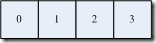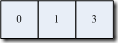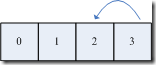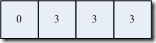# 邵珠庆の博客生命只有一次，你可以用它来做些更多伟大的事情–Make the world a little better and easier

261月/150

## 北京市小汽车摇号程序的反编译、算法及存在的问题浅析-不重复随机数列生成算法

`        public static int[] GetRandomSequence0(int total)`
`        {`
`            int[] hashtable = new int[total];`
`            int[] output = new int[total];`
` `
`            Random random = new Random();`
`            for (int i = 0; i < total; i++)`
`            {`
`                int num = random.Next(0, total);`
`                while (hashtable[num] > 0)`
`                {`
`                    num = random.Next(0, total);`
`                }`
` `
`                output[i] = num;`
`                hashtable[num] = 1;`
`            }`
` `
`            return output;`
`        }``        public static int[] GetRandomSequence1(int total)`
`        {`
`            List<int> input = new List<int>();`
`            for (int i = 0; i < total; i++)`
`            {`
`                input.Add(i);`
`            }`
` `
`            List<int> output = new List<int>();`
` `
`            Random random = new Random();`
`            int end = total;`
`            for (int i = 0; i < total; i++)`
`            {`
`                int num = random.Next(0, end);`
`                output.Add(input[num]);`
`                input.RemoveAt(num);`
`                end--;`
`            }`
` `
`            return output.ToArray();`
`        }`

…… 思考5分钟`        /// <summary>`
`        /// Designed by eaglet`
`        /// </summary>`
`        /// <param name="total"></param>`
`        /// <returns></returns>`
`        public static int[] GetRandomSequence2(int total)`
`        {`
` `
`            int[] sequence = new int[total];`
`            int[] output = new int[total];`
` `
`            for (int i = 0; i < total; i++)`
`            {`
`                sequence[i] = i;`
`            }`
` `
`            Random random = new Random();`
` `
`            int end = total - 1;`
` `
`            for (int i = 0; i < total; i++)`
`            {`
`                int num = random.Next(0, end + 1);`
`                output[i] = sequence[num];`
`                sequence[num] = sequence[end];`
`                end--;`
`            }`
` `
`            return output;`
`        }`

 10000 100000 1000000 GetRandomSequence0 5 44 1075 GetRandomSequence1 11 1038 124205 GetRandomSequence2 1 7 82